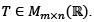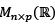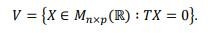Courses

# LetLet V be the subspace ofdefined byThen the dimension of V isa)pn − rank(T)b)mn − p rank(T)c)p(m − rank(T))d)p(n − rank(T))Correct answer is option 'D'. Can you explain this answer? Related Test: Math - 2019 Past Year Paper

## Can you answer this question?

People are searching for an answer to this question.